# Three.js 进阶之旅：神奇的粒子系统-迷失太空 👨‍🚀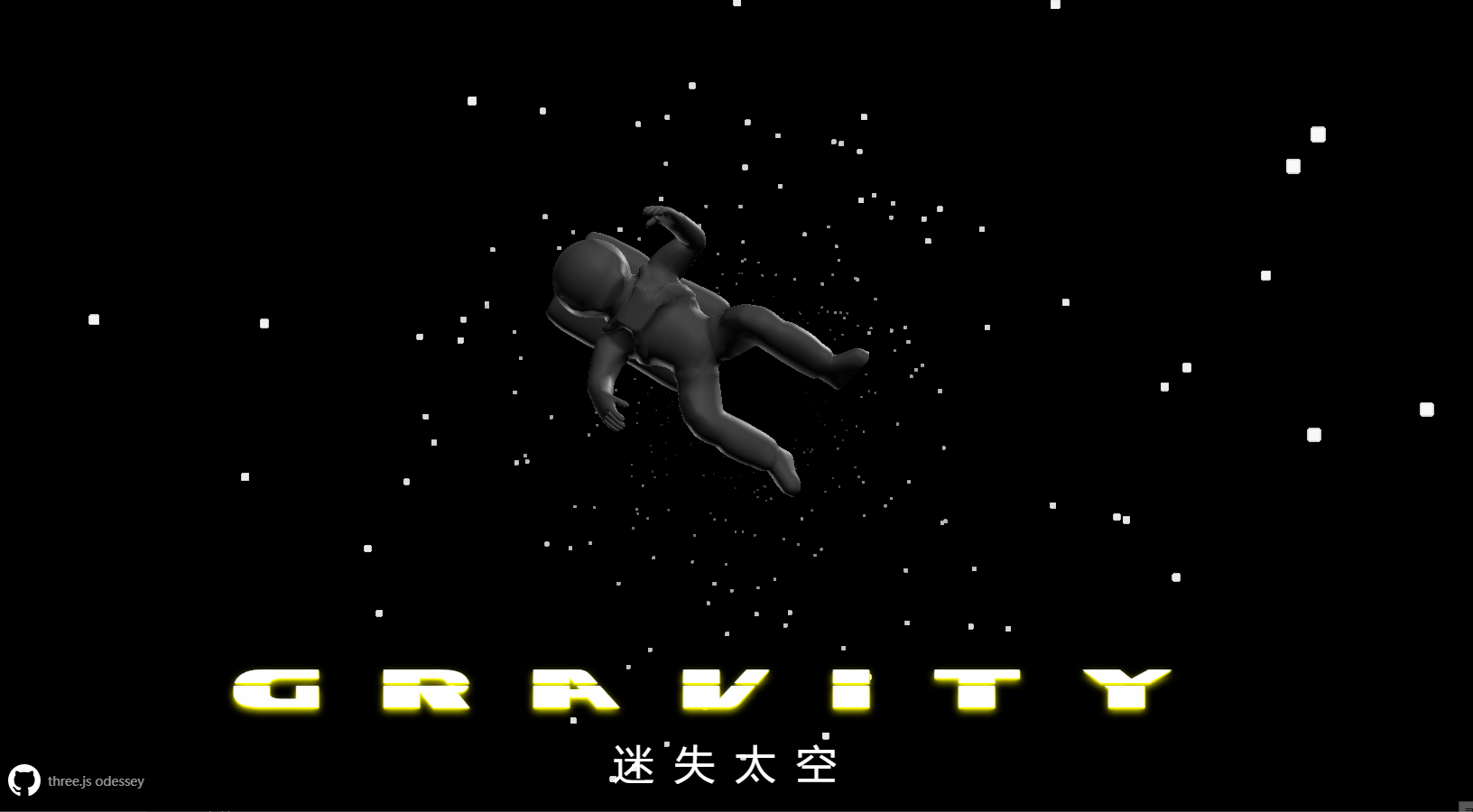## 效果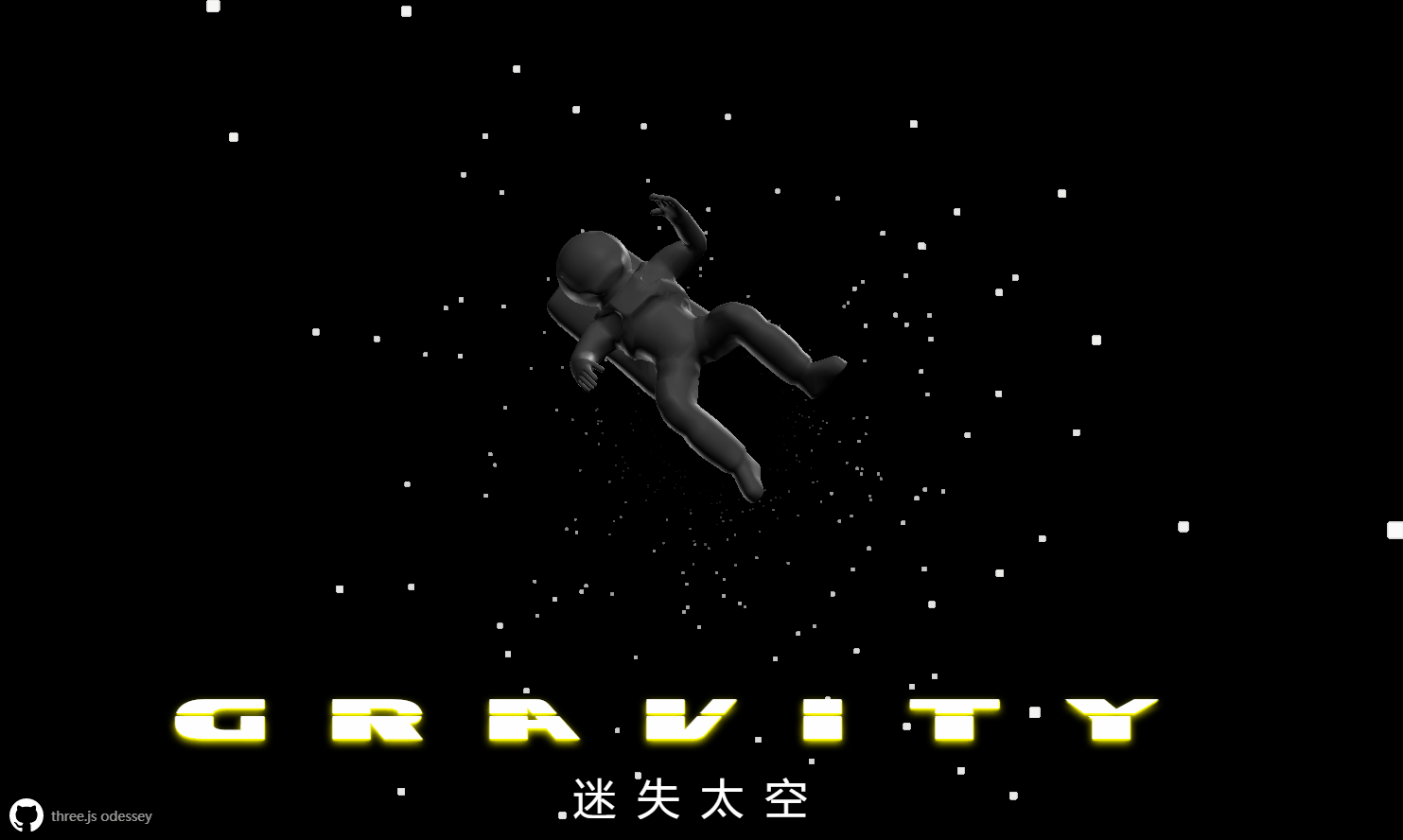`🔗` 代码仓库地址：git@github.com:dragonir/threejs-ode…

《地心引力》海报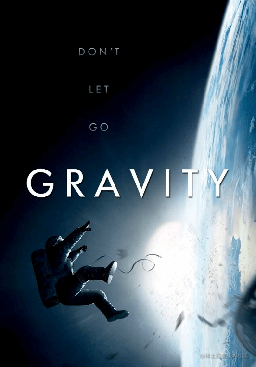## 实现

### 资源引入

``````import './style.css';
import * as THREE from 'three';
import * as dat from 'dat.gui';
import { OrbitControls } from 'three/examples/jsm/controls/OrbitControls';

### 场景初始化

``````// 初始化渲染器
const canvas = document.querySelector('canvas.webgl');
const renderer = new THREE.WebGLRenderer({ canvas: canvas });
renderer.setSize(sizes.width, sizes.height);
renderer.setPixelRatio(Math.min(window.devicePixelRatio, 2));

// 初始化场景
const scene = new THREE.Scene();

// 初始化相机
const camera = new THREE.PerspectiveCamera(45, sizes.width / sizes.height, 0.1, 1000)
camera.position.z = 120
camera.lookAt(new THREE.Vector3(0, 0, 0))

// 镜头控制器
const controls = new OrbitControls(camera, renderer.domElement);
controls.enableDamping = true;

// 页面缩放事件监听
sizes.width = window.innerWidth;
sizes.height = window.innerHeight;
// 更新渲染
renderer.setSize(sizes.width, sizes.height);
renderer.setPixelRatio(Math.min(window.devicePixelRatio, 2))
// 更新相机
camera.aspect = sizes.width / sizes.height;
camera.updateProjectionMatrix();
});

// 页面重绘动画
const tick = () => {
controls && controls.update();
// 更新渲染器
renderer.render(scene, camera);
// 页面重绘时调用自身
window.requestAnimationFrame(tick);
}
tick();

### 〇 使用THREE.Sprite创建粒子

`Three.js` 提供多种方法创建粒子，首先我们使用 `THREE.Sprite` 来通过如下的方式创建一个 `20 x 30` 的粒子系统。通过 `new THREE.Sprite()` 构造方法来创建粒子，给它传入唯一的参数材质，此时可选的材质类型只能是 `THREE.SpriteMaterial``THREE.SpriteCanvasMaterial`。创建材质时将它的 `color` 属性值设置成了随机色。由于`THREE.Sprite` 对象继承于 `THREE.Object3D`，它的大多数属性和方法都可以直接使用。示例中使用了 `position` 方法对粒子进行定位设置。还可以使用 `scale` 属性进行缩放、使用 `translate` 属性进行位移设置等。

``````const createParticlesBySprite = () => {
for (let x = -15; x < 15; x++) {
for(let y = -10; y < 10; y++) {
let material = new THREE.SpriteMaterial({
color: Math.random() * 0xffffff
});
let sprite = new THREE.Sprite(material);
sprite.position.set(x * 4, y * 4, 0);
}
}
}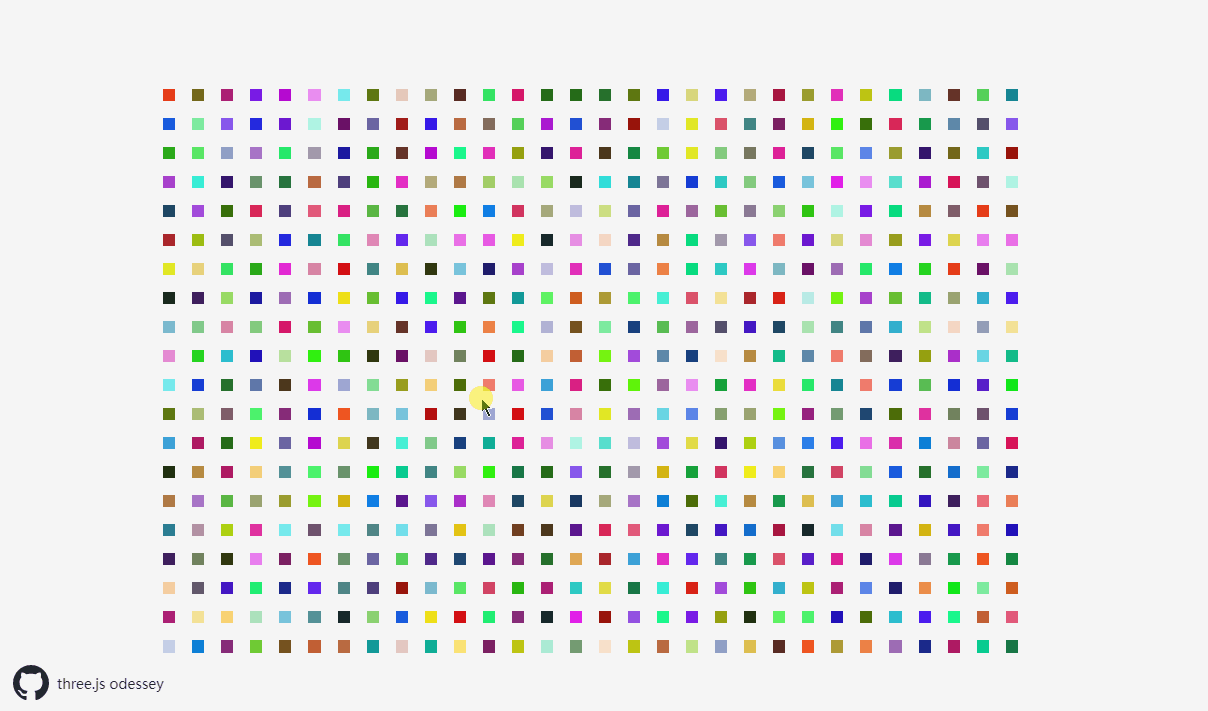#### `知识点 💡` 精灵材质 THREE.SpriteMaterial

`THREE.SpriteMatrial` 对象的一些可修改属性及其说明。

• `color`：粒子的颜色。
• `map`：粒子所用的纹理，可以是一组 `sprite sheet`
• `sizeAttenuation`：如果该属性设置为 `false`，那么距离摄像机的远近不影响粒子的大小，默认值为 `true`
• `opacity`：该属性设置粒子的不透明度。默认值为 `1`，不透明。
• `blending`：该属性指定渲染粒子时所用的融合模式。
• `fog`：该属性决定粒子是否受场景中雾化效果影响。默认值为 `true`

### ① 使用THREE.Points创建粒子

`Three.js` 提供了另一种方式来处理大量的粒子，就是使用 `THREE.Points`，通过 `Three.Points``Three.js` 不需要管理大量 `THREE.Sprite` 对象，而只需要管理 `THREE.Points` 实例。使用这种方法创建粒子系统时，首先要创建粒子的网格 `THREE.BufferGeometry`，然后创建粒子的材质 `THREE.PointsMaterial`。然后创建两个数组 `veticsFloat32Array``veticsColors`，用来管理粒子系统中每个粒子的位置和颜色，通过 `THREE.Float32BufferAttribute` 将它们设置为网格属性。最后使用 `THREE.Points` 将创建的网格和材质变为粒子系统添加到场景中。

``````const createParticlesByPoints = () => {
const geom = new THREE.BufferGeometry();
const material = new THREE.PointsMaterial({
size: 3,
vertexColors: true,
color: 0xffffff
});
let veticsFloat32Array = []
let veticsColors = []
for (let x = -15; x < 15; x++) {
for (let y = -10; y < 10; y++) {
veticsFloat32Array.push(x * 4, y * 4, 0);
const randomColor = new THREE.Color(Math.random() * 0xffffff);
veticsColors.push(randomColor.r, randomColor.g, randomColor.b);
}
}
const vertices = new THREE.Float32BufferAttribute(veticsFloat32Array, 3);
const colors = new THREE.Float32BufferAttribute(veticsColors, 3);
geom.attributes.position = vertices;
geom.attributes.color = colors;
const particles = new THREE.Points(geom, material);
}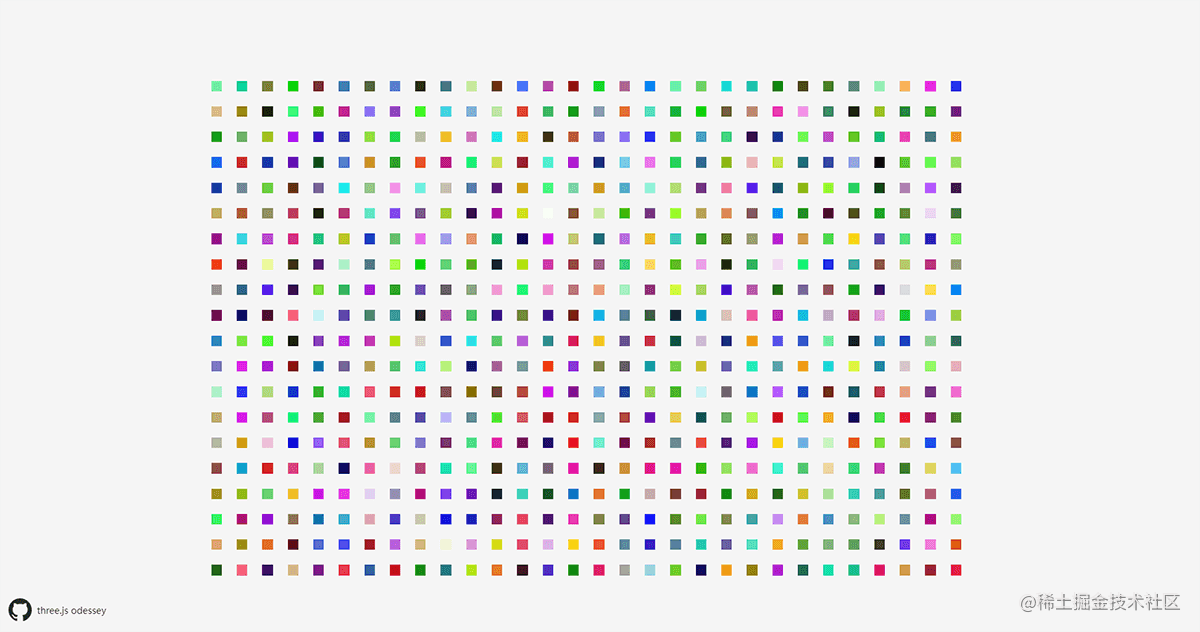#### `知识点 💡` 点材质 THREE.PointsMaterial

• `color`: 粒子系统中所有粒子的颜色。将 `vertexColors` 属性设置为 `true`，并且通过颜色属性指定了几何体的颜色来覆盖该属性。默认值为 `0xFFFFFF`
• `map`: 通过这个属性可以在粒子材质，比如可以使用 `canvas`、贴图等。
• `size`：该属性指定粒子的大小，默认值为 `1`
• `sizeAnnutation`: 如果该属性设置为 `false`，那么所有的粒子都将拥有相同的尺寸，无论它们距离相机有多远。如果设置为 `true`，粒子的大小取决于其距离摄像机的距离的远近，默认值为`true`
• `vertexColors`：通常 `THREE.Points` 中所有的粒子都拥有相同的颜色，如果该属性设置为 `THREE.VertexColors`，并且几何体的颜色数组也有值，那就会使用颜色数组中的值，默认值为 `THREE.NoColors`
• `opacity`：该属性与 `transparent` 属性一起使用，用来设置粒子的不透明度。默认值为 `1`（完全不透明）。
• `transparent`：如果该属性设置为 `true`，那么粒子在渲染时会根据 `opacity` 属性的值来确定其透明度，默认值为 `false`
• `blending`：该属性指定渲染粒子时的融合模式。
• `fog`：该属性决定粒子是否受场景中雾化效果影响，默认值为 `true`

### ② 创建样式化的粒子

``````const createStyledParticlesByPoints = (ctrls) => {
const geom = new THREE.BufferGeometry();
const material = new THREE.PointsMaterial({
size: ctrls.size,
transparent: ctrls.transparent,
opacity: ctrls.opacity,
color: new THREE.Color(ctrls.color),
vertexColors: ctrls.vertexColors,
sizeAttenuation: ctrls.sizeAttenuation
});
let veticsFloat32Array = []
let veticsColors = []
for (let x = -15; x < 15; x++) {
for (let y = -10; y < 10; y++) {
veticsFloat32Array.push(x * 4, y * 4, 0)
const randomColor = new THREE.Color(Math.random() * ctrls.vertexColor)
veticsColors.push(randomColor.r, randomColor.g, randomColor.b)
}
}
const vertices = new THREE.Float32BufferAttribute(veticsFloat32Array, 3)
const colors = new THREE.Float32BufferAttribute(veticsColors, 3)
geom.attributes.position = vertices;
geom.attributes.color = colors;
const particles = new THREE.Points(geom, material);
particles.name = 'particles';
}
// 创建属性控制器
const ctrls = new function () {
this.size = 5;
this.transparent = true;
this.opacity = 0.6;
this.vertexColors = true;
this.color = 0xffffff;
this.vertexColor = 0x00ff00;
this.sizeAttenuation = true;
this.rotate = true;
this.redraw = function () {
if (scene.getObjectByName("particles")) {
scene.remove(scene.getObjectByName("particles"));
}
createStyledParticlesByPoints({
size: ctrls.size,
transparent: ctrls.transparent,
opacity: ctrls.opacity,
vertexColors: ctrls.vertexColors,
sizeAttenuation: ctrls.sizeAttenuation,
color: ctrls.color,
vertexColor: ctrls.vertexColor
});
};
}
const gui = new dat.GUI();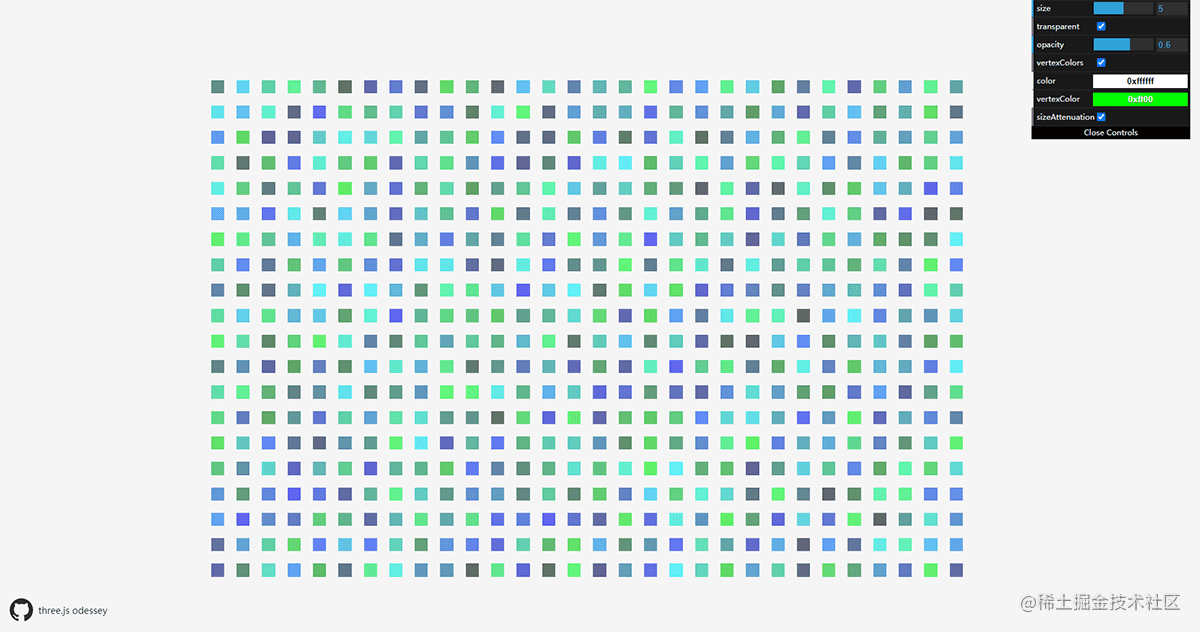#### `知识点 💡` dat.GUI

`dat.GUI` 是一个轻量级的 `JavaScript` 图形用户界面控制库，它可以轻松地即时操作变量和触发函数，通过设定好的控制器去快捷的修改设定的变量。下面是它的一些基本使用方法：

``````// 初始化
const gui = new dat.GUI({ name: 'name'});
// 初始化控件属性
const ctrls = {
name: 'acqui',
speed: 0.5,
color1: '#FF0000',
color2: [0, 128, 255],
color3: [0, 128, 255, 0.3],
color4: { h: 350, s: 0.9, v: 0.3 },
test: ',
test2: ',
cb: () => {},
gender:true
};
// 缩放区间[0,100],变化步长10
// 创建一个下拉选择
gui.add(ctrls, 'test', { 低速: 0.005, 中速: 0.01, 高速: 0.1 }).name('转速');
//  创建按钮
// 控制颜色属性
// 通过name可设置别名
// 创建一个Folder
//可以通过onChange方法来监听改变的值，从而修改样式

`📌` dat.GUI不仅能用到Three.js开发中，在其他需要实时修改参数查看效果的场景下都能用哦。 官网地址：github.com/dataarts/da…

### ③ 使用Canvas样式化粒子

`THREE.js` 提供了将 `HTML5` 画布 `Canvas` 转化为纹理的功能，利用这一特性，我们就可以创建个性化的 `Canvas` 来美化我们的粒子效果。在本文示例 `createParticlesByCanvas` 方法中，首先提供了一个 `createCanvasTexture` 的方法用来生成 `Canvas` 纹理，在该方法中，我们创建一个 `Canvas` 画布，然后在上面绘制一个彩色渐变圆环，最后使用 `THREE.CanvasTexture` 方法将其转化为可以在 `Three.js` 中渲染的纹理。然后使用该纹理，通过 `map` 属性将其传递给粒子材质 `THREE.PointsMaterial`。这样粒子就具有了如下图所示的 `Canvas` 纹理效果了。

``````const createParticlesByCanvas = () => {
// 使用canvas创建纹理
const createCanvasTexture = () => {
const canvas = document.createElement('canvas')
const ctx = canvas.getContext('2d')
canvas.width = 300
canvas.height = 300
ctx.lineWidth = 10;
ctx.beginPath();
ctx.moveTo(170, 120);
var grd = ctx.createLinearGradient(0, 0, 170, 0);
ctx.strokeStyle = grd;
ctx.arc(120, 120, 50, 0, Math.PI * 2);
ctx.stroke();
const texture = new THREE.CanvasTexture(canvas)
texture.needsUpdate = true
return texture
}
// 创建粒子系统
const createParticles = (size, transparent, opacity, sizeAttenuation, color) => {
const geom = new THREE.BufferGeometry()
const material = new THREE.PointsMaterial({
size: size,
transparent: transparent,
opacity: opacity,
map: texture,
sizeAttenuation: sizeAttenuation,
color: color,
depthTest: true,
depthWrite: false
})
let veticsFloat32Array = []
const range = 500
for (let i = 0; i < 400; i++) {
const particle = new THREE.Vector3(Math.random() * range - range / 2, Math.random() * range - range / 2, Math.random() * range - range / 2)
veticsFloat32Array.push(particle.x, particle.y, particle.z)
}
const vertices = new THREE.Float32BufferAttribute(veticsFloat32Array, 3)
geom.attributes.position = vertices
const particles = new THREE.Points(geom, material)
}
createParticles(40, true, 1, true, 0xffffff)
}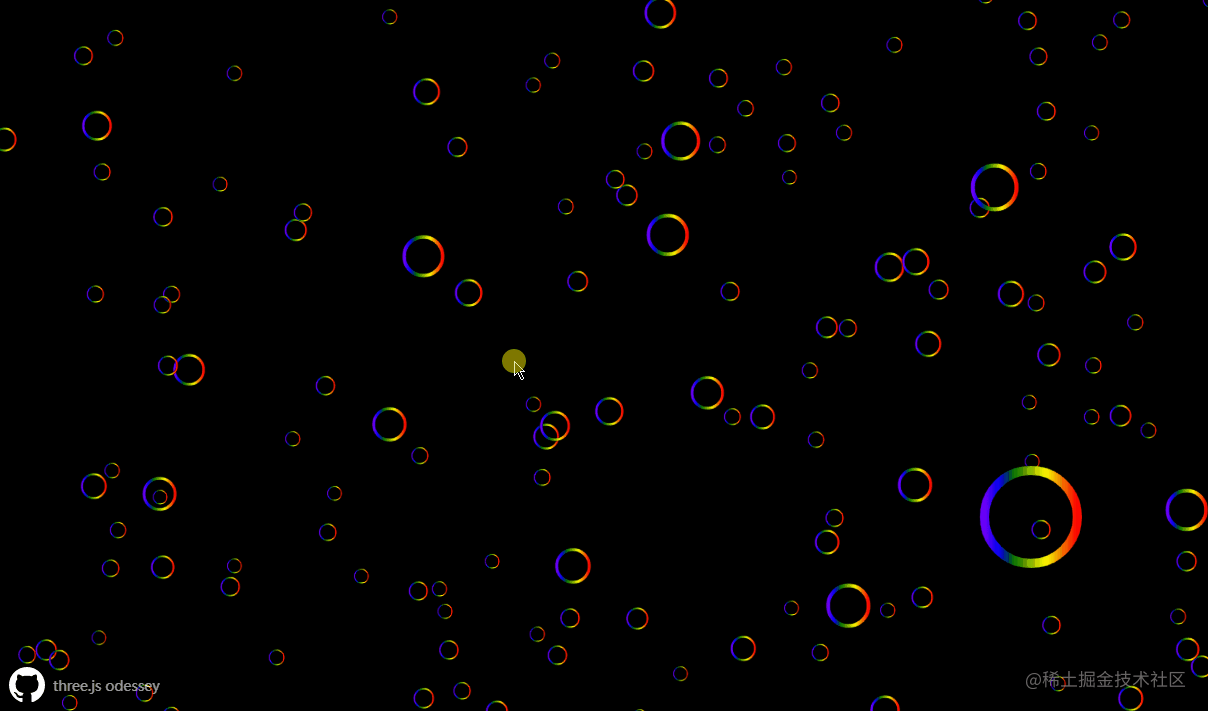#### `知识点 💡` Canvas纹理CanvasTexture

``````CanvasTexture(canvas: HTMLElement, mapping: Constant, wrapS: Constant, wrapT: Constant, magFilter: Constant, minFilter: Constant, format: Constant, type: Constant, anisotropy: Number )

• `canvas`：将会被用于加载纹理贴图的 `Canvas` 元素。
• `mapping`：纹理贴图将被如何应用到物体上。
• `wrapS`：默认值是 `THREE.ClampToEdgeWrapping`
• `wrapT`：默认值是 `THREE.ClampToEdgeWrapping`
• `magFilter`：当一个纹素覆盖大于一个像素时贴图将如何采样，默认值为 `THREE.LinearFilter`
• `minFilter`：当一个纹素覆盖小于一个像素时贴图将如何采样，默认值为 `THREE.LinearMipmapLinearFilter`
• `format`：在纹理贴图中使用的格式。
• `type`：默认值是 `THREE.UnsignedByteType`
• `anisotropy`：沿着轴，通过具有最高纹素密度的像素的样本数。 默认情况下，这个值为 `1`。设置一个较高的值将会产生比基本的 `mipmap` 更清晰的效果，代价是需要使用更多纹理样本。

• `.isCanvasTexture[Boolean]`：检查是否是 `CanvasTexture` 类型纹理的只读属性。
• `.needsUpdate[Boolean]`：是否需要更新，默认值为 `true`，以便使得 `Canvas`中的数据能够载入。
• 其他属性和方法继承于 `Texture`

### ④ 使用纹理贴图样式化粒子

``````const createParticlesByTexture = () => {
const createParticles = (size, transparent, opacity, sizeAttenuation, color) => {
// ...
const material = new THREE.PointsMaterial({
'size': size,
'transparent': transparent,
'opacity': opacity,
// 加载自定义图片作为粒子纹理
'sizeAttenuation': sizeAttenuation,
'color': color,
depthTest: true,
depthWrite: false
})
// ...
}
}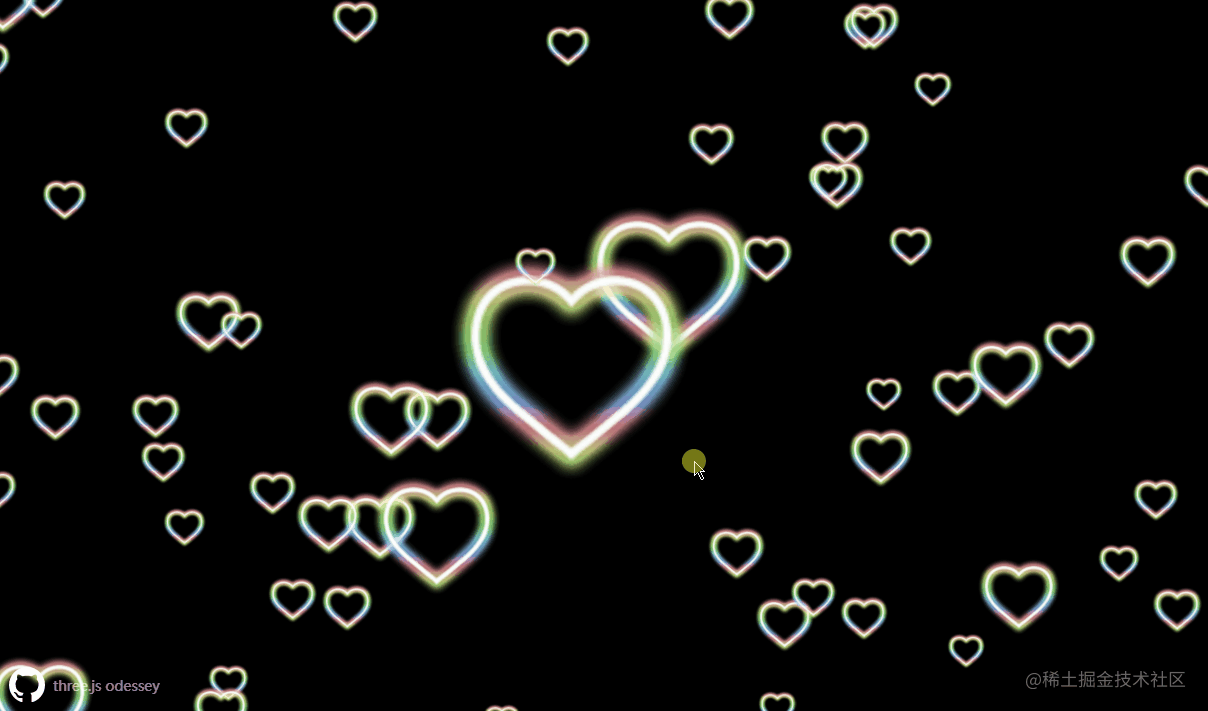### ⑤ 从高级几何体创建粒子

`THREE.Points`基于几何体的顶点来渲染每个粒子的，利用这一特性我们就可以从高级几何体来创建几何体形状的粒子。下面示例中我们利用 `THREE.SphereGeometry` 来创建一个球形的粒子系统。为了营造出好看视觉效果效果，我们可以使用 `Canvas` 的渐变方法 `createRadialGradient` 创建出一种类似发光特效来作为粒子的纹理。

``````const createParticlesByGeometry = () => {
// 创建发光canvas纹理
const generateSprite = () => {
const canvas = document.createElement('canvas');
canvas.width = 16;
canvas.height = 16;
const context = canvas.getContext('2d');
const gradient = context.createRadialGradient(canvas.width / 2, canvas.height / 2, 0, canvas.width / 2, canvas.height / 2, canvas.width / 2);
context.fillRect(0, 0, canvas.width, canvas.height);
const texture = new THREE.Texture(canvas);
texture.needsUpdate = true;
return texture;
}
// 创建立方体
const sphereGeometry = new THREE.SphereGeometry(15, 32, 16);
// 创建粒子材质
const material = new THREE.PointsMaterial({
color: 0xffffff,
size: 3,
transparent: true,
map: generateSprite(),
depthWrite: false
})
const particles = new THREE.Points(sphereGeometry, material)
}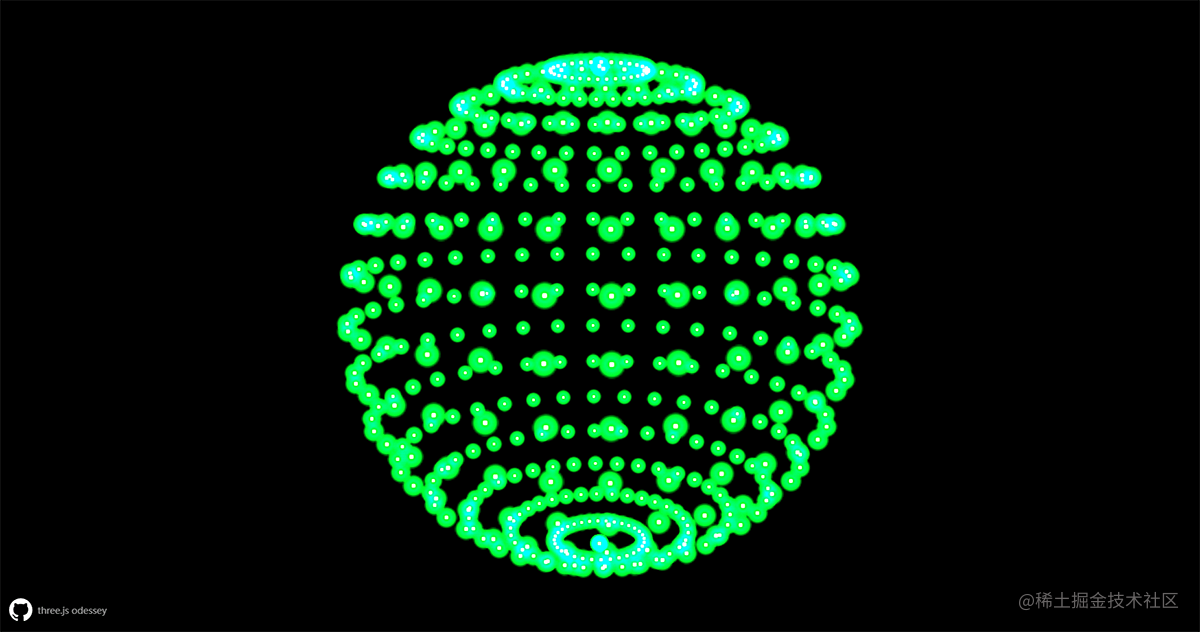### ⑥ 迷失太空

#### 创建宇航员 👨‍🚀

``````const loader = new GLTFLoader();
astronaut = mesh.scene;
astronaut.material = new THREE.MeshLambertMaterial();
astronaut.scale.set(.0005, .0005, .0005);
astronaut.position.z = -10;
});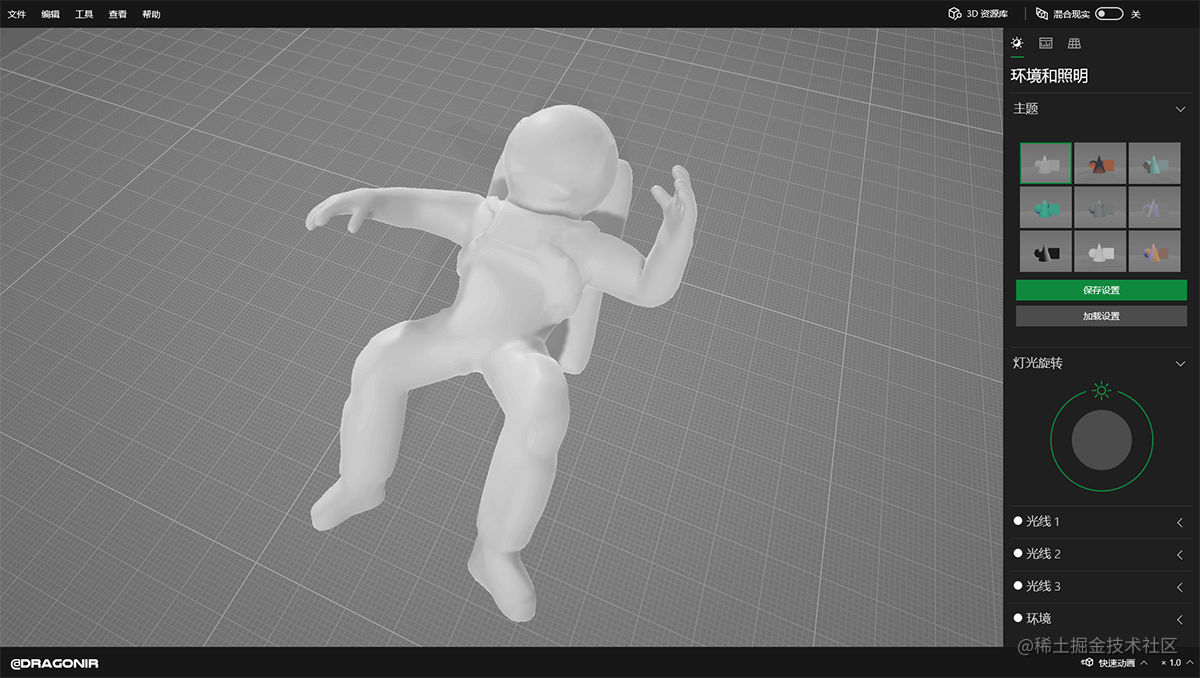#### 创建宇宙粒子 🌌

``````const geom = new THREE.BufferGeometry();
const material = new THREE.PointsMaterial({
color: 0xffffff,
size: 10,
alphaTest: .8,
});
let veticsFloat32Array = []
let veticsColors = []
for (let p = 0; p < 1000; p++) {
veticsFloat32Array.push(
rand(20, 30) * Math.cos(p),
rand(20, 30) * Math.sin(p),
rand(-1500, 0)
);
const randomColor = new THREE.Color(Math.random() * 0xffffff);
veticsColors.push(randomColor.r, randomColor.g, randomColor.b);
}
const vertices = new THREE.Float32BufferAttribute(veticsFloat32Array, 3);
const colors = new THREE.Float32BufferAttribute(veticsColors, 3);
geom.attributes.position = vertices;
geom.attributes.color = colors;
const particleSystem = new THREE.Points(geom, material);

#### 场景优化 ✨

``````// 雾化效果
scene.fog = new THREE.FogExp2(0x000000, 0.005);
// 设置光照
let light = new THREE.PointLight(0xFFFFFF, 0.5);
light.position.x = -50;
light.position.y = -50;
light.position.z = 75;
light = new THREE.PointLight(0xFFFFFF, 0.5);
light.position.x = 50;
light.position.y = 50;
light.position.z = 75;
light = new THREE.PointLight(0xFFFFFF, 0.3);
light.position.x = 25;
light.position.y = 50;
light.position.z = 200;
light = new THREE.AmbientLight(0xFFFFFF, 0.02);

#### `知识点 💡` 雾化效果Fog和FogExp2

• 雾Fog
• 定义：表示线性雾，雾的密度是随着距离线性增大的，即场景中物体雾化效果随着随距离线性变化。
• 构造函数：`Fog(color, near, far)`
• `.color`：表示雾的颜色，场景中远处物体为黑色，场景中最近处距离物体是自身颜色，最远和最近之间的物体颜色是物体本身颜色和雾颜色的混合效果。
• `.near`：表示应用雾化效果的最小距离，距离活动摄像机长度小于 `.near` 的物体将不会被雾所影响。
• `.far`： 表示应用雾化效果的最大距离，距离活动摄像机长度大于 `.far` 的物体将不会被雾所影响。
• 指数雾FogExp2
• 定义：表示指数雾，即雾的密度随着距离指数而增大。
• 构造函数：`FogExp2(color, density)`
• `.color`：表示雾的颜色。
• `.density`：表示雾的密度的增速，默认值为 `0.00025`

#### 添加动画效果 🎦

• 粒子系统动画由以下两部分构成：
• 粒子系统旋转动画：使用余弦函数 `Math.cos(t)` 改变粒子系统的 `position` 来创建旋转运动轨迹，其中 `t` 指定了旋转速度。
• 粒子系统由近到远动画：通过遍历更改构成粒子的向量数组中表示每个粒子的 `Z轴` 方向的值来更新粒子系统实现。
• 宇航员模型动画：
• 宇航员旋转动画：通过修改宇航员在 `x``y``z` 三个坐标轴上的位置参数实现。
• 宇航员由近到远动画：使用正弦函数 `Math.sin(t)` 来生成宇航员远近循环动画。
• 渲染器和相机的页面重绘更新动画。
``````const updateParticles = () => {
// 粒子系统旋转动画
particleSystem.position.x = 0.2 * Math.cos(t);
particleSystem.position.y = 0.2 * Math.cos(t);
particleSystem.rotation.z += 0.015;
camera.lookAt(particleSystem.position);
// 粒子系统由近到远动画
for (let i = 0; i < veticsFloat32Array.length; i++) {
// 如果是Z轴值，则修改数值
if ((i + 1) % 3 === 0) {
const dist = veticsFloat32Array[i] - camera.position.z;
if (dist >= 0) veticsFloat32Array[i] = rand(-1000, -500);
veticsFloat32Array[i] += 2.5;
const _vertices = new THREE.Float32BufferAttribute(veticsFloat32Array, 3);
geom.attributes.position = _vertices;
}
}
particleSystem.geometry.verticesNeedUpdate = true;
}
const updateMeshes = () => {
if (astronaut) {
// 宇航员由近到远动画
astronaut.position.z = 0.08 * Math.sin(t) + (camera.position.z - 0.2);
// 宇航员旋转动画
astronaut.rotation.x += 0.015;
astronaut.rotation.y += 0.015;
astronaut.rotation.z += 0.01;
}
}
// 场景和相机更新
const updateRenderer = () => {
const width = canvas.clientWidth;
const height = canvas.clientHeight;
const needResize = canvas.width !== width || canvas.height !== height;
if (needResize) {
renderer.setSize(width, height, false);
camera.aspect = canvas.clientWidth / canvas.clientHeight;
camera.updateProjectionMatrix();
}
}
const tick = () => {
updateParticles();
updateMeshes();
updateRenderer();
renderer.render(scene, camera);
requestAnimationFrame(tick);
t += 0.01;
}

``````const rand = (min, max) => min + Math.random() * (max - min);

#### 鼠标移动交互 🖱

``````window.addEventListener('mousemove', e => {
const cx = window.innerWidth / 2;
const cy = window.innerHeight / 2;
const dx = -1 * ((cx - e.clientX) / cx);
const dy = -1 * ((cy - e.clientY) / cy);
camera.position.x = dx * 5;
camera.position.y = dy * 5;
astronaut.position.x = dx * 5;
astronaut.position.y = dy * 5;
});

#### CSS样式优化 💥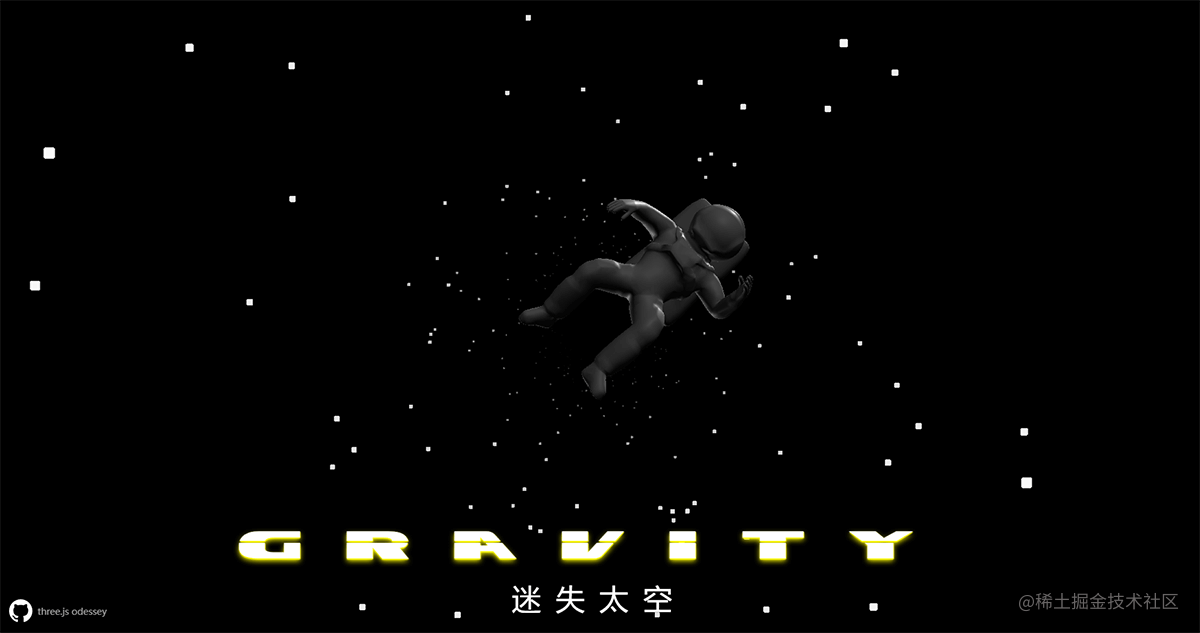## 总结

• 使用 `THREE.Sprite` 创建粒子。
• 使用 `THREE.Points` 创建粒子。
• 创建样式化的粒子。
• 使用 `dat.GUI` 动态控制页面参数。
• 使用 `Canvas` 样式化粒子。
• 使用纹理贴图样式化粒子。
• 从高级几何体创建粒子。
• 给场景添加 `Fog``FogExp2` 雾化效果。
• 使用正弦余弦函数添加模型动画、鼠标移动动画等。